# User:Tohline/Appendix/Ramblings/RiemannB74C692

(Difference between revisions)
 Revision as of 20:45, 28 May 2020 (view source)Tohline (Talk | contribs)← Older edit Revision as of 20:53, 28 May 2020 (view source)Tohline (Talk | contribs) Newer edit → Line 21: Line 21:
- $~\frac{b}{a} = 0.41$ + $~\frac{b}{a} = 0.74$ Line 34: Line 34:
- $~\frac{c}{a} = 0.385$ + $~\frac{c}{a} = 0.692$
- $~\Omega_\mathrm{EFE} = 0.547874$ + $~\Omega_\mathrm{EFE} = 0.638747$ - $~\Omega_\mathrm{EFE} = - 0.079886$ + $~\Omega_\mathrm{EFE} = - 0.217773$
- $~\lambda_\mathrm{EFE} = 0.079886$ + $~\lambda_\mathrm{EFE} = 0.217773$ - $~\lambda_\mathrm{EFE} = - 0.547874$ + $~\lambda_\mathrm{EFE} = - 0.638747$
Line 52: Line 52: Line 61: Line 61:

# Another S-type Example b74c692

This chapter is an extension of the chapter we have titled, "Riemann Meets COLLADA & Oculus Rift S." In that chapter we used as our first example of a Riemann S-type ellipsoid the model with parameters, b41c385. Other closely related chapters are listed below under the heading, "See Also".

## Key Physical Parameters

The model that we have chosen to use in our second successful construction of a COLLADA-based, 3D and interactive animation has the following properties; this model has been selected from Table 2 of our accompanying discussion of Riemann S-type ellipsoids: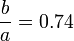$~\frac{b}{a} = 0.74$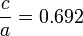$~\frac{c}{a} = 0.692$ Direct Adjoint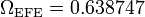$~\Omega_\mathrm{EFE} = 0.638747$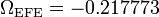$~\Omega_\mathrm{EFE} = - 0.217773$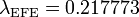$~\lambda_\mathrm{EFE} = 0.217773$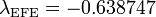$~\lambda_\mathrm{EFE} = - 0.638747$

The subscript "EFE" on Ω and λ means that the relevant frequency is given in units that have been adopted in [EFE], that is, in units of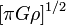$~[\pi G\rho]^{1 / 2}$. In Figure 1a, the solid purple circular marker (where the pair of purple lines cross) identifies the location of this model in the "c/a versus b/a" diagram that appears as Figure 2 on p. 902 of S. Chandrasekhar (1965); essentially the same diagram appears in §49 (p. 147) of [EFE].